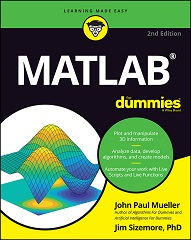﻿ MATLAB For Dummies, 2nd Edition

# MATLAB For Dummies, 2nd EditionPlot graphs, solve equations, and write code in a flash!

If you work in a STEM field, chances are you'll be using MATLAB on a daily basis. MATLAB is a popular and powerful computational tool and this book provides everything you need to start manipulating and plotting your data. MATLAB has rapidly become the premier data tool, and MATLAB For Dummies is a comprehensive guide to the fundamentals. MATLAB For Dummies guides you through this complex computational language from installation to visualization to automation.

• Learn MATLAB's language fundamentals including syntax, operators, and data types.
• Understand how to use the most important window in MATLAB – the Command Window.
• Get the basics of linear algebra to get up and running with vectors, matrices, and hyperspace.
• Automate your work with programming scripts and functions.
• Plot graphs in 2D and 3D to visualize your data.
• Includes a handy guide for MATLAB's functions and plotting routines.

MATLAB is an essential part of the analysis arsenal and MATLAB For Dummies provides clear, thorough guidance to get the most out of your data.

Introduction

#### Part 1: Getting Started With MATLAB

Chapter 1. Introducing MATLAB and Its Many Uses

Chapter 2. Starting Your Copy of MATLAB

Chapter 3. Interacting with MATLAB

Chapter 4. Starting, Storing, and Saving MATLAB Files

#### Part 2: Manipulating and Plotting Data in MATLAB

Chapter 5. Embracing Vectors, Matrices, and Higher Dimensions

Chapter 6. Understanding Plotting Basics

Chapter 7. Using Advanced Plotting Features

#### Part 3: Streamlining MATLAB

Chapter 9. Expanding MATLAB’s Power with Functions

Chapter 11. Working with Live Scripts

Chapter 12. Working with Live Functions

Chapter 13. Designing and Using Classes

Chapter 14. Creating MATLAB Apps

Chapter 15. Building Projects

#### Part 4: Employing Advanced MATLAB Techniques

Chapter 16. Importing and Exporting Data

Chapter 17. Printing and Publishing Your Work

Chapter 18. Recovering from Mistakes

#### Part 5: Specific MATLAB Applications

Chapter 19. Solving Equations and Finding Roots

Chapter 20. Performing Analysis

#### Part 6: The Part of Tens

The Top Ten Uses of MATLAB

Chapter 1. Ten Ways to Make a Living Using MATLAB

Appendix A: MATLAB Functions

Appendix B: MATLAB’s Plotting Routines

If you find an error or have any questions, please email us at admin@erenow.org. Thank you!